# Massive Number Calculator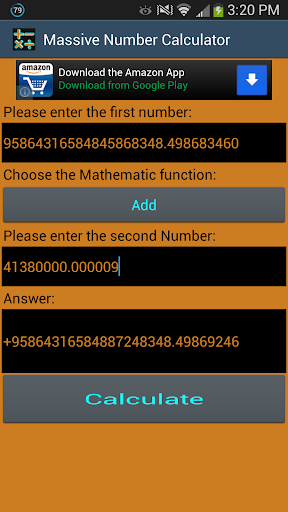This calculator can perform simple mathematical functions for up to 2.1 Billion digits long, and will give the result of a calculation in full length! Addition options found in the menu include: Clear All, and Input Pi. You may input Pi to however many digits you like, but be careful, the more digits, the longer the wait!

The mathematical functions include: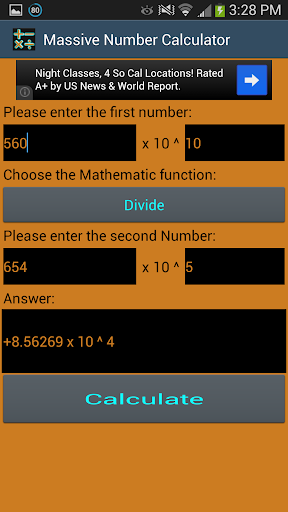* Add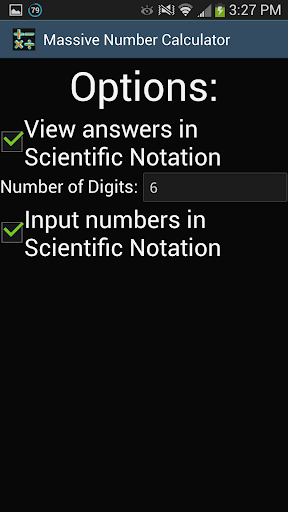* Subtract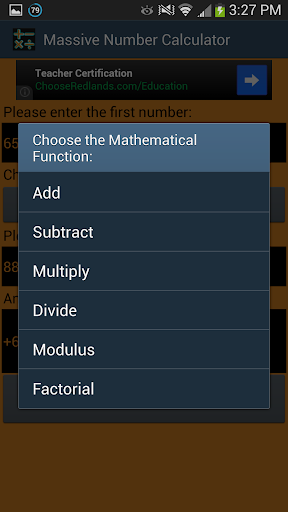* Multiply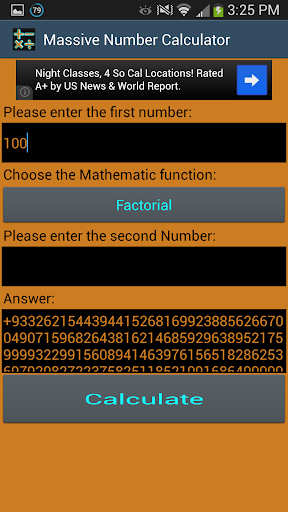* Divide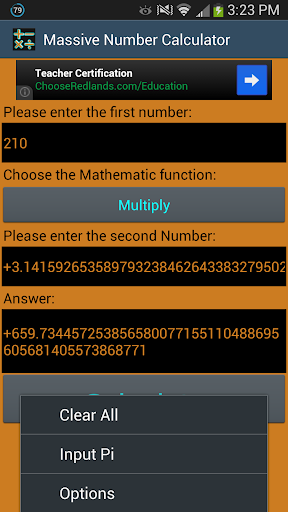* Modulus

* Factorial

### 免費玩Massive Number Calculator APP玩免費

#### 免費玩Massive Number Calculator App

Google Play
1.3
App下載

1970-01-012015-04-22